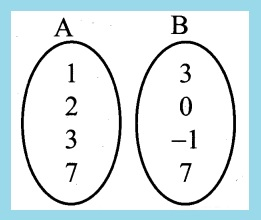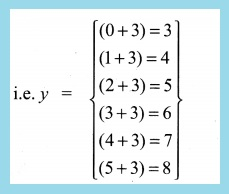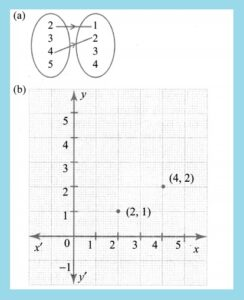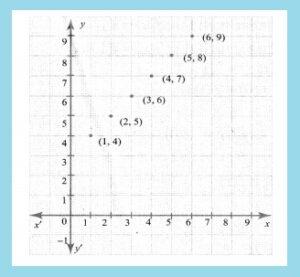State Tamil Nadu Board State Board [Samacheer Kalvi] Class 10th/SSLC Subject Maths [English Medium] Download Guide/ Solution/ Book Answers Chapter Chapter 1 Relations and Functions Exercise Exercise 1.2

## Samacheer Kalvi Class 10th Maths Chapter 1 Ex 1.2 Book Answers

Samacheer Kalvi Class 10th Maths Chapter 1 Relations and Functions Exercise 1.2 Answers

Question 1:
Let A = {1, 2, 3, 7} and B = {3, 0, -1, 7}, which of the following are relation from A to B ?

(i) R1 = {(2, 1), (7, 1)}
(ii) R2 = {(-1, 1)}
(iii) R3 = {(2, -1), (7, 7), (1, 3)}
(iv) R4 = {(7,-1), (0, 3), (3, 3), (0, 7)}
(i) A = {1, 2, 3, 7}, B = {3, 0,-1, 7}

Solution:
R1 = {(2,1), (7,1)}It is not a relation there is no element as 1 in B.
(ii) R2 = {(-1, 1)}
It is not [∵ -1 ∉ A, 1 ∉ B]
(iii) R3 = {(2, -1), (7, 7), (1, 3)}
It is a relation.
R4 = {(7,-1), (0, 3), (3, 3), (0, 7)}
It is also not a relation. [∵ 0 ∉ A]

———————————————————————————————————————————————————————————————————————————————————————————————————————————————-

Question 2:
Let A = {1, 2, 3, 4, ….., 45} and R be the relation defined as “is square of ” on A. Write R as a subset of A × A. Also, find the domain and range of R.

A = {1,2, 3, 4 . . . . 45}
The relation is defined as “is square of’
R = {(1,1) (2, 4) (3, 9)
(4, 16) (5,25) (6, 36)}
Domain of R = {1, 2, 3, 4, 5, 6}
Range of R = {1, 4, 9, 16, 25, 36}

———————————————————————————————————————————————————————————————————————————————————————————————————————————————-

Question-3:
A Relation R is given by the set {(x, y) /y = x + 3, x ∈ {0, 1, 2, 3, 4, 5}}. Determine its domain and range.
Solution:
x = {0, 1, 2, 3, 4, 5}
y = x + 3⇒ y = {3, 4, 5, 6, 7, 8}
R = {(x, y)}
= {(0, 3),(1, 4),(2, 5),(3, 6), (4, 7), (5, 8)}
Domain of R = {0, 1, 2, 3, 4, 5}
Range of R = {3, 4, 5, 6, 7, 8}

———————————————————————————————————————————————————————————————————————————————————————————————————————————————-

Question-4:
Represent each of the given relation by (a) an arrow diagram, (b) a graph and (c) a set in roster form, wherever possible.
(i) {(x, y)|x = 2y,x ∈ {2, 3, 4, 5},y ∈ {1, 2, 3, 4)
(ii) {(x, y)|y = x + 3, x, y are natural numbers <10}

Solution:
(i){(x, y)|x = 2y, x ∈ {2, 3, 4, 5},y ∈ {1, 2, 3, 4}} R = (x = 2y)
2 = 2 × 1 = 2
4 = 2 × 2 = 4(c) {(2, 1), (4, 2)}
(ii) {(x, y)|y = x + 3, x,+ are natural numbers <10}
x = {1, 2, 3, 4, 5, 6, 7, 8, 9} R = (y = x + 3)
y = {1, 2, 3, 4, 5, 6, 7, 8, 9}
R = {(1, 4), (2, 5), (3, 6), (4, 7), (5, 8), (6, 9)}

b)(c) R = {(1, 4), (2, 5), (3, 6), (4, 7), (5, 8), (6, 9)}

———————————————————————————————————————————————————————————————————————————————————————————————————————————————-

Question-5:
A company has four categories of employees given by Assistants (A), Clerks (C), Managers (M) and an Executive Officer (E). The company provide ₹10,000, ₹25,000, ₹50,000 and ₹1,00,000 as salaries to the people who work in the categories A, C, M and E respectively. If A1, A2, A3, A4 and As were Assistants; C1, C2, C3, C4 were Clerks; M1, M2, M3 were managers and E1,E2 were Executive officers and if the relation R is defined by xRy, where x is the salary given to person y, express the relation R through an ordered pair and an arrow diagram.

Solution:
A – Assistants → A1, A2, A3, A4, A5
C – Clerks → C1, C2, C3, C4
D – Managers → M1, M2, M3
E – Executive officer → E1, E2
(a) R = {(10,000, A1), (10,000, A2), (10,000, A3),
(10,000, A4), (10,000, A5), (25,000, C1),
(25,000, C2), (25,000, C3), (25,000, C4),
(50,000, M1), (50,000, M2), (50,000, M3),
(1,00,000, E1), (1,00,000, E2)}

———————————————————————————————————————————————————————————————————————————————————————————————————————————————-

samacheer kalvi 10th standard maths guide, samacheer kalvi 10th maths answer key, class 10 samacheer kalvi english solutions, class 10th maths guide samacheer kalvi, samacheer kalvi class 10th maths guide answers, samacheer kalvi class 10th maths guide answer key, samacheer kalvi class 10th maths guide app, samacheer kalvi class 10th maths guide all formulas, samacheer kalvi class 10th maths guide algebra important questions, samacheer kalvi class 10th maths guide answers solutions guide, maths samacheer kalvi guide 10th, maths guide for class 10 samacheer kalvi pdf, maths samacheer kalvi 10th, maths samacheer kalvi books 10th answers, samacheer kalvi class 10th maths guide book, samacheer kalvi class 10th maths guide book pdf, samacheer kalvi class 10th maths guide book back answers, samacheer kalvi class 10th maths guide book back answers solutions, samacheer kalvi class 10th maths guide books free download pdf, samacheer kalvi class 10th maths guide books english, samacheer kalvi class 10th maths guide bank

Have a question? Please feel free to reach out by leaving a comment below

(Visited 75 times, 1 visits today)